# Angles, Polygons and Geometrical Proof - Stage 3### Square It

##### Age 11 to 16Challenge Level

Players take it in turns to choose a dot on the grid. The winner is the first to have four dots that can be joined to form a square.### Square Coordinates

##### Age 11 to 14Challenge Level

A tilted square is a square with no horizontal sides. Can you devise a general instruction for the construction of a square when you are given just one of its sides?### Triangles in Circles

##### Age 11 to 14Challenge Level

Can you find triangles on a 9-point circle? Can you work out their angles?### Subtended Angles

##### Age 11 to 14Challenge Level

What is the relationship between the angle at the centre and the angles at the circumference, for angles which stand on the same arc? Can you prove it?### Right Angles

##### Age 11 to 14Challenge Level

Can you make a right-angled triangle on this peg-board by joining up three points round the edge?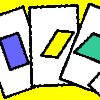##### Age 11 to 14Challenge Level

A game for 2 or more people, based on the traditional card game Rummy.### Shapely Pairs

##### Age 11 to 14Challenge Level

A game in which players take it in turns to turn up two cards. If they can draw a triangle which satisfies both properties they win the pair of cards. And a few challenging questions to follow...### Property Chart

##### Age 11 to 14Challenge Level

A game in which players take it in turns to try to draw quadrilaterals (or triangles) with particular properties. Is it possible to fill the game grid?### Semi-regular Tessellations

##### Age 11 to 16Challenge Level

Semi-regular tessellations combine two or more different regular polygons to fill the plane. Can you find all the semi-regular tessellations?##### Age 11 to 16Challenge Level

Draw some quadrilaterals on a 9-point circle and work out the angles. Is there a theorem?### Which Solids Can We Make?

##### Age 11 to 14Challenge Level

Interior angles can help us to work out which polygons will tessellate. Can we use similar ideas to predict which polygons combine to create semi-regular solids?### Opposite Vertices

##### Age 11 to 14Challenge Level

Can you recreate squares and rhombuses if you are only given a side or a diagonal?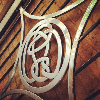##### Age 11 to 14Challenge Level

How many questions do you need to identify my quadrilateral?##### Age 11 to 14Challenge Level

We started drawing some quadrilaterals - can you complete them?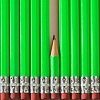### Angles, Polygons and Geometrical Proof Short Problems

##### Age 11 to 16

A collection of short problems on Angles, Polygons and Geometrical Proof.### Of All the Areas

##### Age 14 to 16Challenge Level

Can you find a general rule for finding the areas of equilateral triangles drawn on an isometric grid?### Estimating Angles

##### Age 7 to 14Challenge Level

How good are you at estimating angles?### Tilted Squares

##### Age 11 to 14Challenge Level

It's easy to work out the areas of most squares that we meet, but what if they were tilted?### Hidden Squares

##### Age 11 to 14Challenge Level

Can you find the squares hidden on these coordinate grids?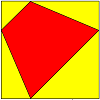##### Age 11 to 14Challenge Level### Polygon Rings

##### Age 11 to 14Challenge Level

Join pentagons together edge to edge. Will they form a ring?### Star Polygons

##### Age 11 to 14Challenge Level

Draw some stars and measure the angles at their points. Can you find and prove a result about their sum?### Polygon Pictures

##### Age 11 to 14Challenge Level

Can you work out how these polygon pictures were drawn, and use that to figure out their angles?### An Equilateral Triangular Problem

##### Age 11 to 14Challenge Level

Take an equilateral triangle and cut it into smaller pieces. What can you do with them?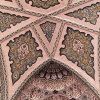### Angles Inside

##### Age 11 to 14Challenge Level

Draw some angles inside a rectangle. What do you notice? Can you prove it?